Disclosure: This post may contain affiliate links, meaning when you click the links and make a purchase, we receive a commission.

# How to Calculate Variance in Excel

In this article, we shall show you how to calculate variance in Excel. Variance is one of the most useful concepts in statistics. It gives the measurement of how the numbers of data are distributed about the mean/expected value. It measures the distribution by looking at all the data points.

Normally variance is the difference between an expected and actual result. In statistics, the variance is calculated by dividing the square of the deviation about the mean with the number of population. To calculate the deviation about the mean the difference of each individual value with the arithmetic mean is taken and then all the differences are summed up. Here in this article, we will analyze different methods and functions for calculating variance in excel.

## How to Calculate Percentage Variance in Excel

To calculate percentage variance, we can use the formula Variance = (new value-original value)/original value. This will give you a decimal number. After formatting this into percentage format you will get the result as a percentage. You don’t need to multiply the result by 100. The following picture shows the result of normal variance. If the original value is greater than the new value you will get a negative result. To avoid the negative result, use the function ABS in the formula. It makes the negative number positive. So the formula will become, Variance = ABS(new value-original value)/original value.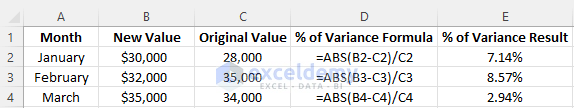## How to Calculate Population Variance in Excel With Formula

For calculating the population variance, the following formula is used.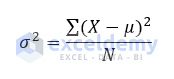Here,

• μ is the arithmetic mean
• X is the individual value
• N is the size of the population
•  is the population variance

Let`s say we want to calculate the population variance of five data which have different values. For easier calculation make two columns which are for (X-μ) and (X-μ)2 .Now for calculating the total number of data use the formula =COUNT(B2: B6). The resultant value will be N for this formula. For calculating the arithmetic mean for the individual values use the formula =AVERAGE (B2: B6). Use the formula =B2-\$B\$10 in cell C2 and drag the formulated cell downwards to copy this formula for the whole column to calculate the deviation about the mean (X-μ). To square, the deviation about the mean (X-μ) in a different column, use the formula =C2^2 and drag the formulated cell to copy the formula for the whole column. Then calculate the sum of the square of the deviation about the mean (X-μ)2 using the formula =SUM (D2: D6). Then finally calculate the population variance by dividing the square of the deviation about the mean (X-μ)2 by the total number of data. Here we found the result 82.16. The following picture shows the result and necessary data table for the calculation.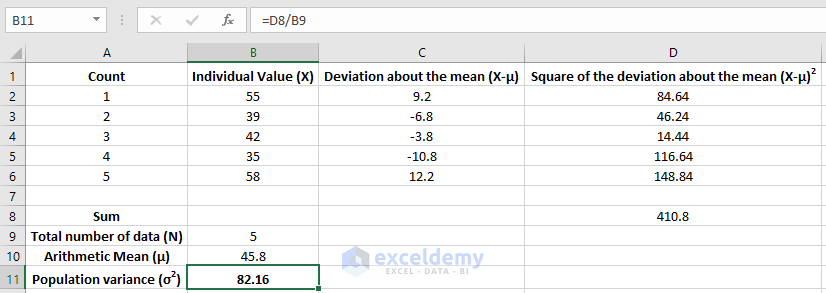## How to Calculate Sample Variance in Excel With Formula

For calculating the population variance, the following formula is used.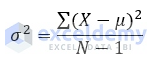Here,

• μ is the arithmetic mean
• X is the individual value
• N is the size of the population
•  is the population variance

Here we will use the same example for the calculation. As you can see, there is only one difference between the previous formula and this. It is that the sample formula has a denominator of N-1. Previously the denominator was N. The whole calculation in Excel will be same. The only difference will be dividing the square of the deviation about the mean (X-μ)2 by (N-1) instead of N. Here the calculated value is 102.7. Instead of 5, the deviation about the mean (X-μ)2 is now divided by 4. So, with the same values of data, the sample variance is always greater than the population variance. The following picture shows the result and necessary data table for the calculation of sample variance.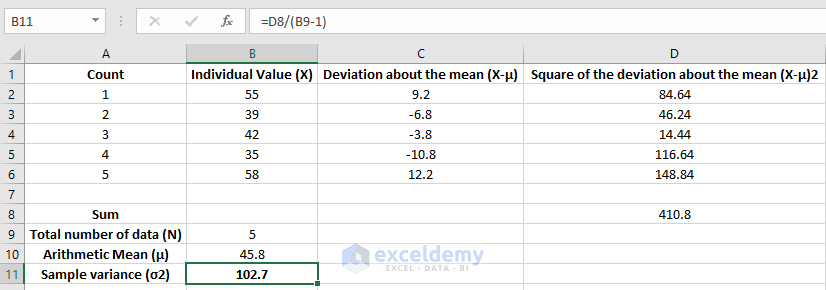## Calculating Population Variance & Sample Variance with Built-in Function in Excel

The above description describes the detail calculation process of sample and population variance. In excel there are 4 built-in functions for calculating the variances. You just need to define the range for which you want to find the variance.

Suppose you want to calculate the population variance using the excel built-in function. Here we will be using the same example to see if our result matches with previous population variance result or not. The function that we will be using is VAR.P. This function gives the variance from a whole population. Put the range inside the brackets to perform the population variance just like this, =VAR.P(B2: B6). The following picture shows the population variance with VAR.P function.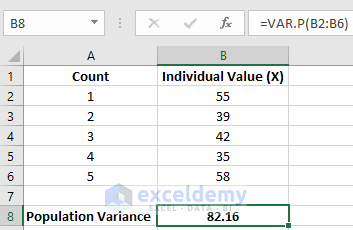To show the sample variance you can use the function VAR.S. After putting the function define the range within the brackets just like this =VAR.S(B2: B6). The following picture shows the result of sample variance with VAR.S function.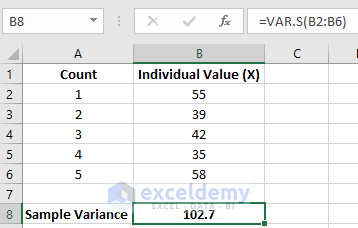There are other two functions to calculate the variance. They are VARA and VARPA. In VAR.P and VAR.S functions, the logical and text values are ignored. But VARA and VARPA evaluate the logical and text values in the calculation. The following picture shows the 4 functions together where different results are found.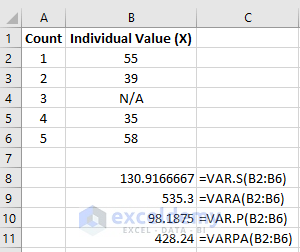VARA evaluates the simple variance whereas VARPA evaluates the population variance. The Excel 2007 and its earlier version don’t have these functions. They have VAR and VARP functions where the VAR calculate the sample variance and VARP calculates the variance based on an entire population.

## Conclusion

For proper visualization of statistical data, it is important to measure the variance. Without the variance, you can not measure the standard deviation. In this article, I showed different approaches for calculating the variance in excel. Following the step by step process for calculating the variance is a longer process of calculation. There are many functions for calculating the variance and these are faster than the step by step calculations.

You will find all the calculations provided in this article in the below file.

How to calculate Variance in Excel-calculation

I hope you will find this article useful. Follow this website for many useful articles regarding Microsoft Excel.